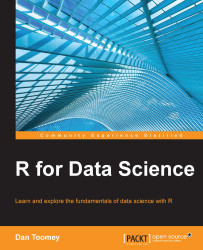•#### R for Data Science#### Overview of this book

R for Data ScienceCreditswww.PacktPub.comPrefaceFree Chapter
Data Mining PatternsData Mining SequencesText MiningData Analysis – Regression AnalysisData Analysis – CorrelationData Analysis – ClusteringData Visualization – R GraphicsData Visualization – PlottingData Visualization – 3DMachine Learning in ActionPredicting Events with Machine LearningSupervised and Unsupervised LearningIndex## Chapter 5. Data Analysis – Correlation

Correlation is a good technique to use on your dataset. Correlation helps us to establish basic relationships between data items.

In this chapter, we look at tools available in R for correlation:

• A basic correlation

• Visualizing correlations

• Covariance

• Pearson correlation

• Polychoric correlation

• Tetrachoric correlation

• A heterogeneous correlation matrix

• Partial correlation